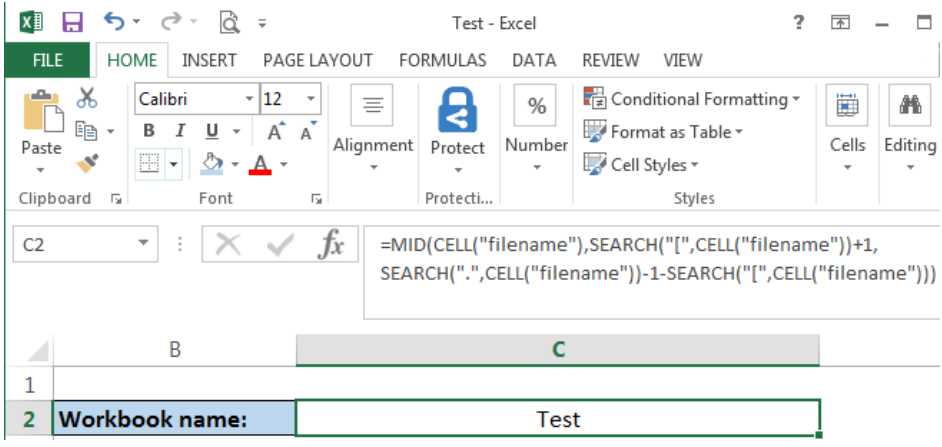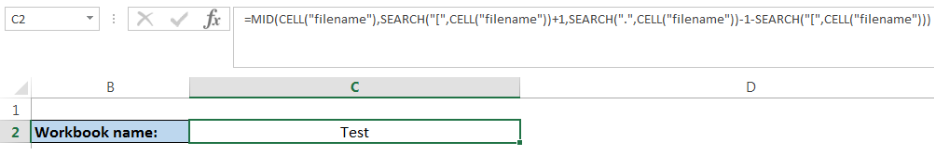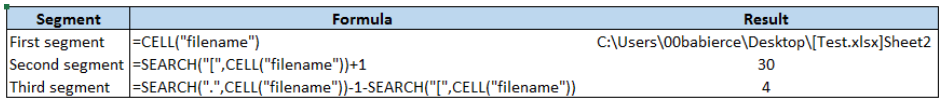Get instant live expert help with Excel or Google Sheets“My Excelchat expert helped me in less than 20 minutes, saving me what would have been 5 hours of work!”

#### Post your problem and you’ll get Expert help in seconds.

Your message must be at least 40 characters
Our professional Expert are available now. Your privacy is guaranteed.

# Get workbook name only

Excel allows us to obtain the workbook name of the file we are working on, by using the CELL, MID and SEARCH functions.  The CELL function provides information about a cell such as the cell’s address, format or contents.  The MID function extracts a substring from the middle of a string while the SEARCH function returns the starting position of a substring within a string. This step by step tutorial will assist Excel users in getting only the workbook name of an Excel file.Figure 1. Final result : Get the workbook name of the Excel file “Test”

Final formula: `=``MID(CELL("filename"),SEARCH("[",CELL("filename"))+1,SEARCH(".",CELL("filename"))-1-SEARCH("[",CELL("filename")))`

## Syntax of the CELL function

`=CELL(info_type, [reference])`

where

• info_type – a text which specifies the type of information about the cell that we want to obtain; allowed values are: address, col (for column), color, contents, filename, format, parentheses, prefix, protect, row, type, width
• reference optional; the cell that we want to obtain information about; if omitted, the reference cell is the cell that was last modified

## Syntax of the MID function

`=MID(text, start_num, num_chars)`

where

• text – the text string containing the substring we want to obtain
• start_num – the position of the first character of the substring we want to obtain
• num_chars – the number of characters we want MID to return

## Syntax of the SEARCH function

`=SEARCH(find_text, within_text, [start_num])`

• find_text – the substring that we want to find
• within_text -the text string where we will search for the value of the find_text argument
• start_num optional; the position of the character in the within_text argument where we want to start searching

## Get workbook name only

We want to get only the name of the workbook.

This is the working formula using the CELL, MID and SEARCH functions:

`=MID(CELL("filename"),SEARCH("[",CELL("filename"))+1,``SEARCH(".",CELL("filename"))-1-SEARCH("[",CELL``("filename")))`Figure 2. Entering the formula to get the workbook name

To apply the formula, we follow these steps:

• Select cell C2
• Insert the formula: `=``MID(CELL("filename"),SEARCH("[",CELL("filename"))+1,SEARCH(".",CELL("filename"))-1-SEARCH("[",CELL("filename")))`
• Press enterFigure 3. Result for the workbook name: “Test”

The formula returns the workbook name: “Test”.

## Explanation

Working formula:

`=MID(CELL("filename"),SEARCH("[",CELL("filename"))+1,``SEARCH(".",CELL("filename"))-1-SEARCH("[",CELL``("filename")))`

To understand the formula, we divide the MID formula into three segments.

First segment : `CELL("filename")`

Second segment: `SEARCH("[",CELL("filename"))+1`

Third segment: `SEARCH(".",CELL("filename"))-1-SEARCH("[",CELL("filename")) `Figure 4. Arguments for the MID formula to get the workbook name

Remember that the syntax for the MID function is:

`=MID(text, start_num, num_chars)`

• The first segment serves as the text – which returns the cell filename C:\Users\00babierce\Desktop\[Test.xlsx]Sheet2
• The second segment is the argument for start_num – the position of the first character of the workbook name “Test” ; in this case, start_num is 30 or the 30th character of the cell filename
• The third segment is the argument for num_chars – the number of characters we want MID to return, which is 4 (Test has 4 characters)

Most of the time, the problem you will need to solve will be more complex than a simple application of a formula or function. If you want to save hours of research and frustration, try our live Excelchat service! Our Excel Experts are available 24/7 to answer any Excel question you may have. We guarantee a connection within 30 seconds and a customized solution within 20 minutes.

Solution examplesI need Vlookup help across 2 workbooks into one other main workbook and can't figure out what i'm doing wrong!
Solved by G. E. in 19 minsI need a formula .... i need to decrease the premium an employee pays by 10% each year for ages 65-75. So when they are 65 it decreases 10%, age 66 it decreases 20% and so on. I tried =IF(OR(\$D2+1<65,\$D2+1=66,\$D2+2=77, ...... ),J2,J2-(J2*0.1),J2(J2*0.2) .... ) D2 being the current age of the employee, with each column being year 1, year 2, etc. However, excel will not allow me to do to IF D2+1=66, til age 75. It's too long. I know there has to be a better way! HELP!
Solved by F. F. in 60 minsI need to total a column of values that are in a custom format h:mm:ss; when you total it in a number format. If I change it to the same customer format I then need to deduct if from total hours of production. and does not look right I am wondering if my total hours worked which is a number value should be changed to look correct
Solved by K. W. in 60 minsI am not sure if my vlookup is working correctly. I have 30,000 ID to match but only 3,000 matched which makes me concern. I need to identify the duplicates and eliminate them.
Solved by V. E. in 60 minsI have a column where each cell has letters and numbers. The numbers would range from 10 through 18. I would like to find a formula where I can search the cell for any one of the numbers and return a value from a different cell if it finds one. I currently search for a specific number and the formula works but only for that particular number. The formula I currently have is: =IF(ISNUMBER(SEARCH("16",F10)),G10,"") This does work if it finds the value of 16 in the (F10)cell and then places the value from cell "G10" as the result. If it doesn't find the numbers, it will leave the resultant cell blank.
Solved by O. H. in 60 mins